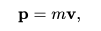# Unit of Momentum

The most common terminology we have heard is “gaining momentum”, this is said when the sports players perform well and if the game is progressing. Similarly, the term momentum refers to the quantity of motion associated with the body. Momentum is defined as the product of mass and velocity. It is a vector quantity which has both magnitude and direction.In Newtonian mechanics, translational momentum, linear momentum, or simply momentum is the product of the mass and velocity of an object.  Momentum of the body depends on the mass and velocity. If either of the two values is zero, then the momentum becomes zero.

In reality, there is no specific name for the unit of momentum. This is because momentum is a product of mass and force and expressed in the units of these quantities. Besides, if we look at the formula of momentum it is given as;

So, considering this condition, we will look at the possible units below.

 Momentum SI unit kilogram meter per second (kg⋅m/s) Common symbols p, p Other units slug⋅ft/s Dimension MLT−1

## SI Unit of Momentum

As mentioned above, the units of momentum will be the product of the units of mass and velocity. Mass is measured in kg and velocity in ms-1, therefore, the SI unit of momentum will be kgm/s(-1).

### Other Momentum Units

If we consider the situation when force is equivalent to the rate of change of momentum: i.e. Force=(change in momentum)/(time interval) then, change in momentum = Force * (time interval). Therefore, the unit for momentum can be Newton-second (Ns).

In the CGS system, if the mass is in grams and the velocity in centimeters per second, then the unit of momentum will be gram-centimeters per second (g⋅cm/s).

Stay tuned with BYJU’S for more such interesting articles. Also, register to “BYJU’S – The Learning App” for loads of interactive, engaging Physics-related videos and an unlimited academic assist.

Related Articles:

Test your Knowledge on unit of moment